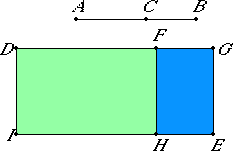# Proposition 75

If from a medial straight line there is subtracted a medial straight line which is commensurable with the whole in square only, and which contains with the whole a medial rectangle, then the remainder is irrational; let it be called second apotome of a medial straight line.
X.28

From the medial straight line AB let there be subtracted the medial straight line CB which is commensurable with the whole AB in square only such that the rectangle AB by BC which it contains with the whole AB, is medial.

I say that the remainder AC is irrational, and let it be called a second apotome of a medial straight line.II.7

Set out a rational straight line DI. Apply DE, equal to the sum of the squares on AB and BC, to DI producing DG as breadth. Apply DH, equal to twice the rectangle AB by BC, to DI producing DF as breadth. Then the remainder FE equals the square on AC.

Now, since the squares on AB and BC are medial and commensurable, therefore DE is also medial.

X.22

And it is applied to the rational straight line DI, producing DG as breadth, therefore DG is rational and incommensurable in length with DI.

X.23,Cor.

Again, since the rectangle AB by BC is medial, therefore twice the rectangle AB by BC is also medial.

And it equals DH, therefore DH is also medial.

X.22

And it is applied to the rational straight line DI, producing DF as breadth, therefore DF is rational and incommensurable in length with DI.

X.11

Since AB and BC are commensurable in square only, therefore AB is incommensurable in length with BC. Therefore the square on AB is also incommensurable with the rectangle AB by BC.

But the sum of the squares on AB and BC is commensurable with the square on AB, and twice the rectangle AB by BC is commensurable with the rectangle AB by BC, therefore twice the rectangle AB by BC is incommensurable with the sum of the squares on AB and BC.

But DE equals the sum of the squares on AB and BC, and DH equals twice the rectangle AB by BC, therefore DE is incommensurable with DH. But DE is to DH as GD is to DF, therefore GD is incommensurable with DF.

X.73

And both are rational, therefore GD and DF are rational straight lines commensurable in square only. Therefore FG is an apotome.

X.20

But DI is rational, and the rectangle contained by a rational and an irrational straight line is irrational, and its side is irrational.

And AC is the side of FE, therefore AC is irrational.

Let it be called a second apotome of a medial straight line.

Q.E.D.

## Guide

This proposition is used for a few later propositions in Book X starting with X.81.